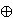### Pavle Pandžić

Department of Mathematics, University of Zagreb, Bijenička 30, 10 000 Zagreb, Croatia
e-mail: pandzic@math.hr

Abstract.   Let G be a connected real reductive Lie group with a maximal compact subgroup K corresponding to a Cartan involution Θ of G. Let q=lu be a θ-stable parabolic subalgebra of the complexified Lie algebra g of G, where θ=dΘ. Let L be the centralizer of q in G. We show that, under certain dominance assumptions, cohomological induction with respect to q takes irreducible unitary (l, L ∩ K)-modules with nonzero Dirac cohomology to irreducible unitary (g,K)-modules which also have nonzero Dirac cohomology.

2000 Mathematics Subject Classification.   22E47.

Key words and phrases.   Reductive Lie group, unitary representation, Harish-Chandra module, Dirac operator, Dirac cohomology, cohomological induction, bottom layer.

Full text (PDF) (free access)

DOI: 10.3336/gm.45.2.12

References:

1. C. Chevalley, The algebraic theory of spinors, Columbia University Press, 1954.
MathSciNet

2. T. J. Enright, R. Howe and N. R. Wallach, A classification of unitary highest weight modules, in Representation theory of reductive groups, Park City, Utah, 1982, Birkhäuser, Boston, 1983, 97-143.
MathSciNet

3. J.-S. Huang, Y.-F. Kang and P. Pandžić, Dirac cohomology of some Harish-Chandra modules, Transform. Groups 14 (2009), 163-173.
MathSciNet     CrossRef

4. J.-S. Huang and P. Pandžić, Dirac cohomology, unitary representations and a proof of a conjecture of Vogan, J. Amer. Math. Soc. 15 (2002), 185-202.
MathSciNet     CrossRef

5. J.-S. Huang and P. Pandžić, Dirac operators in representation theory, Mathematics: Theory and Applications, Birkhauser, 2006.
MathSciNet

6. J.-S. Huang, P. Pandžić and V. Protsak, Dirac cohomology of Wallach representations, preprint, 2009.

7. J.-S. Huang, P. Pandžić and D. Renard, Dirac operators and Lie algebra cohomology, Represent. Theory 10 (2006), 299-313.
MathSciNet     CrossRef

8. A. W. Knapp and D. A. Vogan, Cohomological induction and unitary representations, Princeton University Press, 1995.
MathSciNet

9. B. Kostant, Clifford algebra analogue of the Hopf-Koszul-Samelson theorem, the ρ-decomposition C(g)=End Vρ⊗ C(P), and the g-module structure of ⋀ g, Adv. Math. 125 (1997), no. 2, 275-350.
MathSciNet     CrossRef

10. B. Kostant, A cubic Dirac operator and the emergence of Euler number multiplets of representations for equal rank subgroups, Duke Math. J. 100 (1999), 447-501.
MathSciNet     CrossRef

11. R. Parthasarathy, Dirac operator and the discrete series, Ann. of Math. 96 (1972), 1-30.
MathSciNet     CrossRef

12. K. R. Parthasarathy, R. Ranga Rao and V. S. Varadarajan, Representations of complex semisimple Lie groups and Lie algebras, Ann. of Math. 85 (1967), 383-429.
MathSciNet     CrossRef

13. D. A. Vogan, Representations of real reductive Lie groups, Birkhäuser, Boston, 1981.
MathSciNet

14. D. A. Vogan, Dirac operators and unitary representations, 3 talks at MIT Lie groups seminar, Fall 1997.

15. D. A. Vogan, G. J. Zuckerman, Unitary representations with non-zero cohomology, Compositio Math. 53 (1984), 51-90.
MathSciNet     CrossRef

16. N. R. Wallach, Real Reductive Groups, Volume I, Academic Press, 1988.
MathSciNet